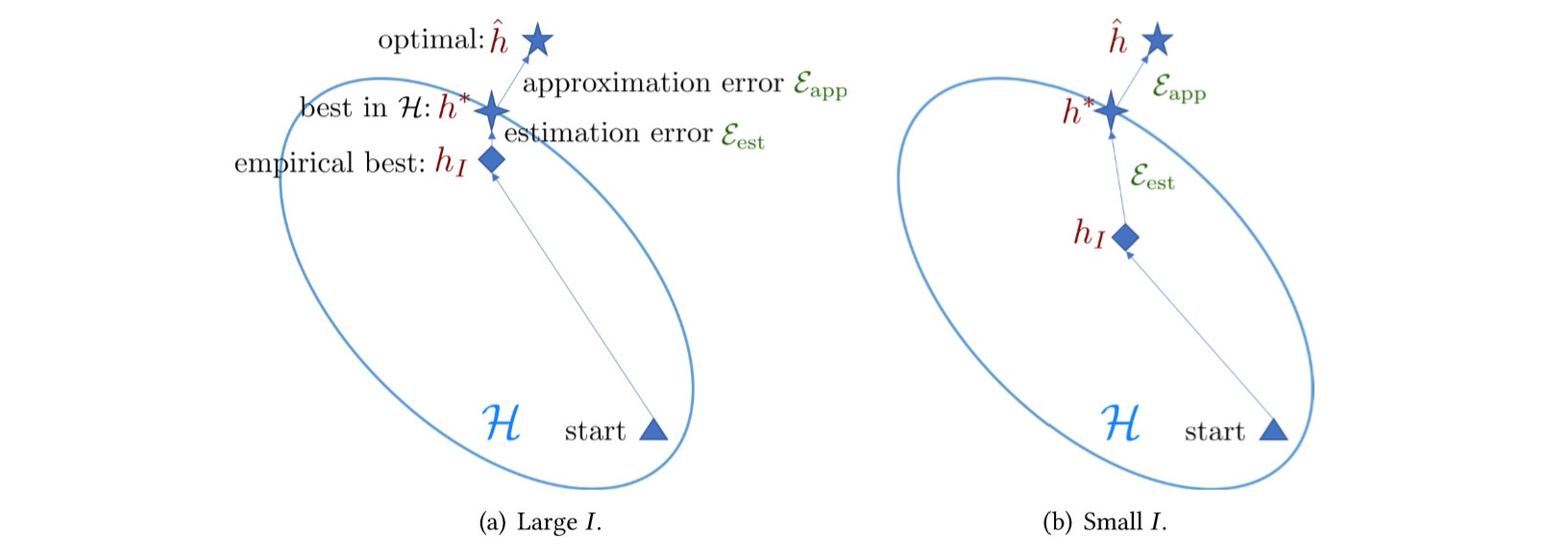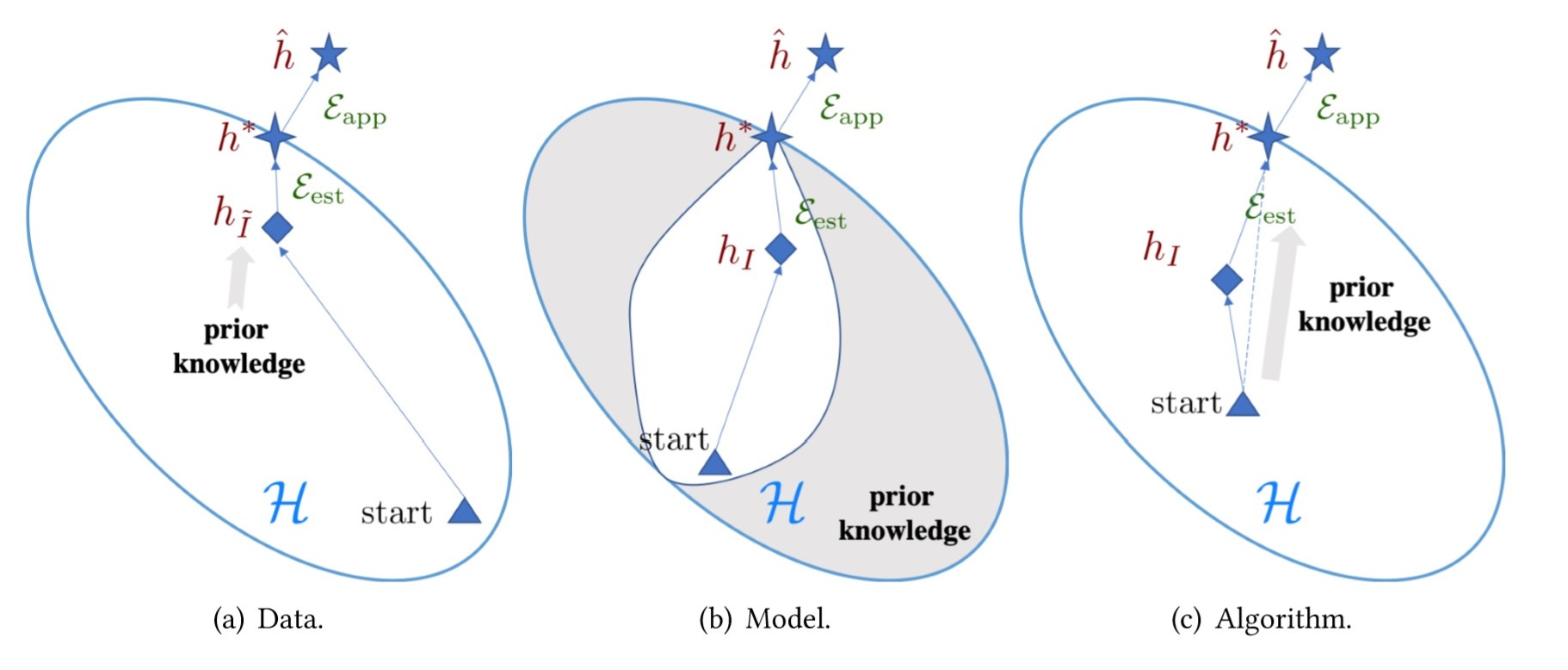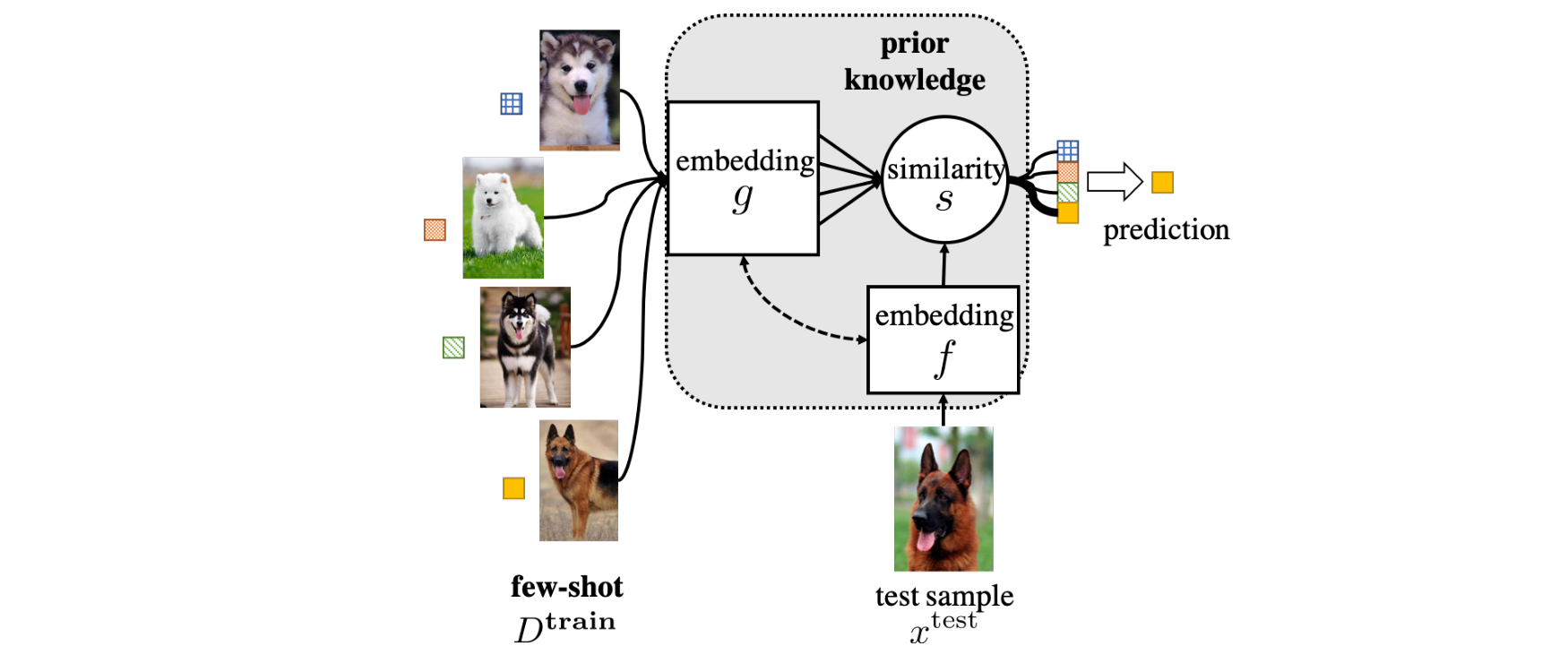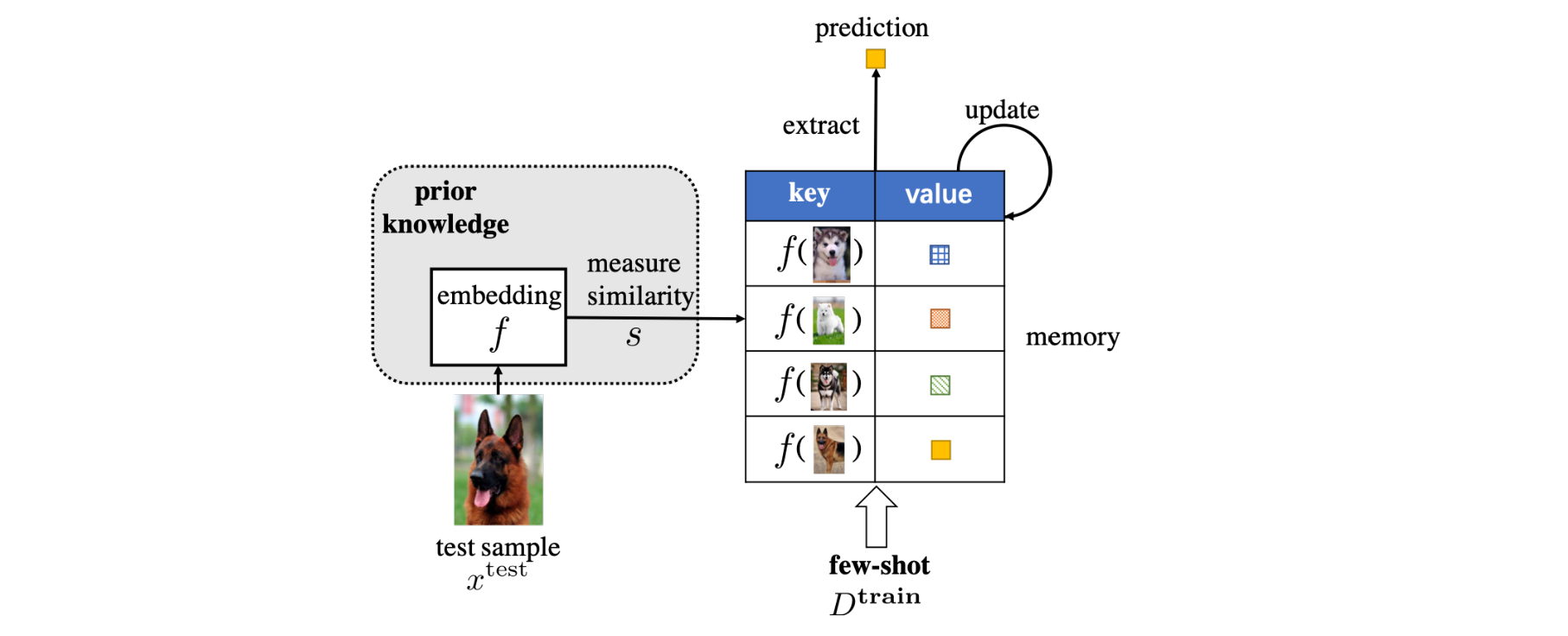Jarvis' Blog (总有美丽的风景让人流连) 总有美丽的风景让人流连

# 小样本学习: 基础 (Few-Shot Learning: Basic)

2019-08-10
Jarvis
Post

1. 数据增广
2. 缩小模型的假设空间
3. 设计更好的优化算法

## 1. 引言

FSL 有许多使用场景. (1) 用于测试 AI 程序是否能像人那样做出决策. (2) 帮助工业界减轻收集大规模训练数据的负担, 比如 ResNet2 在 1000 类图像分类数据集上获得了人类水平的表现, 但该数据集包含了百万张图像及标注. (3) 因为某些原因, 数据集的监督信息难以获得的情况, 如医学图像的标注需要专家进行, 药品研发的过程中可能产生毒性等等. 这些因素使得收集大量的有标注数据时困难的.

• Few-Shot Learning Survey3

## 2. 基本概念

### 2.1 问题定义

FSL 是机器学习的一个子领域, 因此我们首先回顾机器学习的定义.

Definition 1. (Machine Learning4) A computer program is said to learn from experience $$E$$ with respect to some classes of task $$T$$ and performance measure $$P$$ if its performance can improve with $$E$$ on $$T$$ measured by $$P$$ .

FSL 是一种特殊的机器学习任务, 这类任务只能使用少量的监督信息构成训练数据集. FSL 的定义如下:

Definition 2. (Few-Shot Learning) A type of machine learning problem(specified by $$E, T$$ and $$P$$) where $$E$$ contains a little supervised information for the target $$T$$.

### 2.2 相关问题

• 半监督学习(Semi-supervised Learning) 是同时从有标注和无标注的数据中学习最优假设 $$\hat{h}$$ . 正样本半监督学习(Positive-unlabeled learning) 是一类特殊的半监督学习, 只有正样本和无标注样本可以使用. 主动学习(active learning) 则由算法选择可以提供有用信息(informative)的无标注数据由专家给出标注, 反馈给模型学习. 所以 FSL 既可以是监督学习, 也可以是半监督学习, 取决于从有限的监督信息中可以获得哪种数据.
• 不平衡学习(Imbalance Learning) 从类别 $$y$$ 的分布严重倾斜的数据集中学习. FSL 任务中每类数据本来就很少.
• 迁移学习(Transfer Learning) 是把知识从数据量丰富的源域迁移到数据量不足的目标域. 领域自适应(domain adaption) 就是一种迁移学习问题. 另一种相关的迁移学习叫零样本学习(zero-shot learning), 它需要在已有类别中学习特征, 并根据已有特征的组合来判断新的从未见过的类别. 根据 FSL 的定义, FSL 不一定是迁移学习, 但当我们使用额外监督信息来提升目标数据集的表现时, 这本质上就是一种迁移学习, 只不过源域中的数据可能也不多.
• 元学习(Meta Learning)/学会学习(Learning to Learn) 通过提供的数据集和元学习器(meta-learner)从其他地方学到的知识在新的任务 $$T$$ 上提升表现 $$P$$ . 具体来说, 元学习器在许多任务中学习元信息(一般知识), 并能够使用任务相关的信息快速泛化到新的任务上. 许多 FSL 都是元学习方法, 使用元学习器作为先验信息.

### 2.3 核心问题

$\min_{\theta}\sum_{(x^{(u)}, y^{(i)})\in D^{train}}l(h(x^{(i)};\theta), y),$

#### 2.3.1 经验风险最小化

$R(h)=\int l(h(x), y)dp(x, y)=\mathbb{E}[l(h(x), y)].$

$R_I(h)=\frac1n\sum_{i=1}^Il(h(x^{(i)}), y),$

• $$\hat{h}=\arg\min_fR(h)$$, 表示 $$\hat{h}$$ 是 $$R$$ 的全局最优解
• $$h^\*=\arg\min_{h\in\mathcal{H}}R(h)$$, 表示 $$h^*$$ 是 $$R$$ 在假设空间 $$\mathcal{H}$$ 中的最优解
• $$h_I=\arg\min_{h\in\mathcal{H}}R_I(h)$$, 表示 $$h_I$$ 是 $$R_I$$ 在假设空间 $$\mathcal{H}$$ 中的最优解

$\mathbb{E}[R(h_I)-R(\hat{h})]=\underbrace{\mathbb{E}[R(h^*)-R(\hat{h})]}_{\displaystyle\mathcal{E}_{app}(\mathcal{H})}+\underbrace{\mathbb{E}[R(h_I)-R(h^*)]}_{\displaystyle\mathcal{E}_{est}(\mathcal{H, I})},$

#### 2.3.2 不可靠的经验风险最小化

$\lim_{I\rightarrow\infty}\mathcal{E}_{est}(\mathcal{H, I})=\lim_{I\rightarrow\infty}\mathbb{E}[R(h_I)-R(h^*)]=0,$#### 2.3.3 FSL 方法的分类

FSL 必须使用先验信息来辅助任务的解决, 因此根据先验信息的不同使用方法可以分为三类:## 3. 基于数据的方法

### 3.1 训练集 $$D^{train}$$ 数据增广

#### 3.1.1 人工规则

• 平移 (translating)
• 翻转 (flipping)
• 错切 (shearing)
• 缩放 (scaling)
• 镜像 (reflecting)
• 裁剪 (cropping)
• 旋转 (rotating)
• 加噪 (noising)
• 亮度 (birghtness)
• 对比度 (contrast)
• 饱和度 (saturation)

(注: 后期增加各类框架的API)

## 4. 基于模型的方法

multitask learning 其他任务 $$T$$ 及相应的数据集 $$D$$ 参数共享
embedding learning 从/和其他任务 $$T$$ 学到的嵌入 特征降维, 在低维嵌入空间中分类
learning with external memory 从其他任务 $$T$$ 学到的嵌入与记忆交互
generative modeling 从其他任务学到的先验模型 限制分布的形式

• 硬 (Hard) 参数共享
• 共享神经网络前几层参数, 最后几层适配不同任务 (Fine-Grained Visual Categorization5).
• 共享某些层, 源任务更新共享层的参数和特有层的参数, 目标任务只更新其特有层的参数 (One-Shot Unsupervised Cross Domain Translation7).
• 软 (Soft) 参数共享 软共享不进行参数的强约束, 只鼓励不同任务的参数尽可能满足某种条件 (比如使用正则化函数 $$L_1, L_2$$ 约束不同任务参数的距离). 因此多任务参数软共享时, 不同任务仍然具有不同的假设空间和参数.

### 4.2 嵌入学习 (Embedding Learning)

• 测试数据的嵌入函数 $$f(\cdot)$$
• 训练样本的嵌入函数 $$g(\cdot)$$
• 度量 $$s(\cdot, \cdot)$$• Matching Network 通过计算 $$f(x^{test})$$ 和一系列 $$g(x_i), x_i\in D^{sup}$$ 的相似度来对 $$x^{test}$$ 进行分类, 其中 $$f(\cdot)$$ 依赖于 $$D^{train}$$ 而 $$g(\cdot)$$ 使用双向 LSTM (biLSTM) 聚合了 $$D^{sup}$$ 中所有样本的信息. 在 Matching Nets 中两个嵌入函数为不同的模型, 度量使用了余弦相似度 (cosine similarity).

• Prototypical Network 对支撑集中每一类的样本计算一个原型 (prototype, $$c$$) 用来代表该类别的嵌入. 原型用该类别中有标签样本的嵌入的平均值表示, 即

$c_n = \frac1K\sum_{k=1}^K g(x_k^{(n)}),$

其中 $$n$$ 表示第 $$n$$ 个类别. 在 Prototypical Nets 中, 两个嵌入函数使用了相同的模型, 度量使用了 $$L_2$$ 距离.

• Relative Representations 进一步使用模型来学习度量. 如 Relation Network 使用 CNN 首先把数据映射到嵌入空间, 然后把测试数据和支撑数据的嵌入并起来, 通过另一个神经网路预测相似度值.

• Relation Graph 把支撑集和测试集的样本点作为图节点, 边权则通过学习得到. 测试集的分类任务利用邻近节点的信息进行预测.

### 4.3 外部记忆学习 (Learning with External Memory)

1. 记忆检索相关的信息
2. 计算相似度
3. 记忆可以被更新## 参考文献

1. One-Shot Learning of Object Categories
Li Fei-Fei, R. Fergus, and P. Perona.
[link]. In IEEE Transactions on Pattern Analysis and Machine Intelligence 28 (4): 594–611. 2006.

2. Deep Residual Learning for Image Recognition
He, Kaiming, Xiangyu Zhang, Shaoqing Ren, and Jian Sun.
[link]. In Proceedings of the IEEE Conference on Computer Vision and Pattern Recognition, 770–78. 2016.

3. Generalizing from a Few Examples: A Survey on Few-Shot Learning
Wang, Yaqing, Quanming Yao, James Kwok, and Lionel M. Ni.
[link] In ArXiv:1904.05046 [Cs], April. 2019.

4. Machine Learning Textbook
Tom Mitchell.

5. Fine-grained visual categorization using meta-learning optimization with sample selection of auxiliary data
Yabin Zhang, Hui Tang, Kui Jia